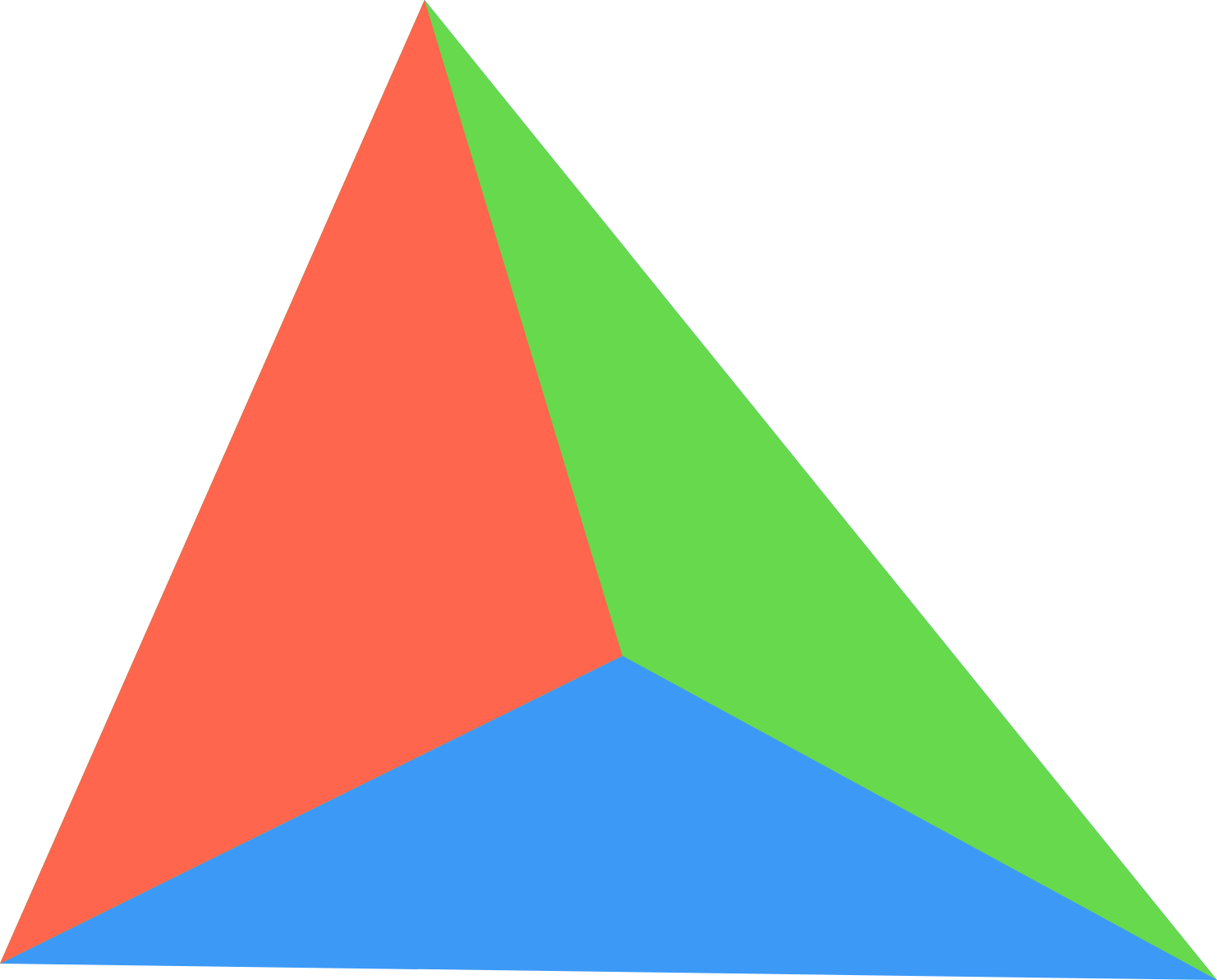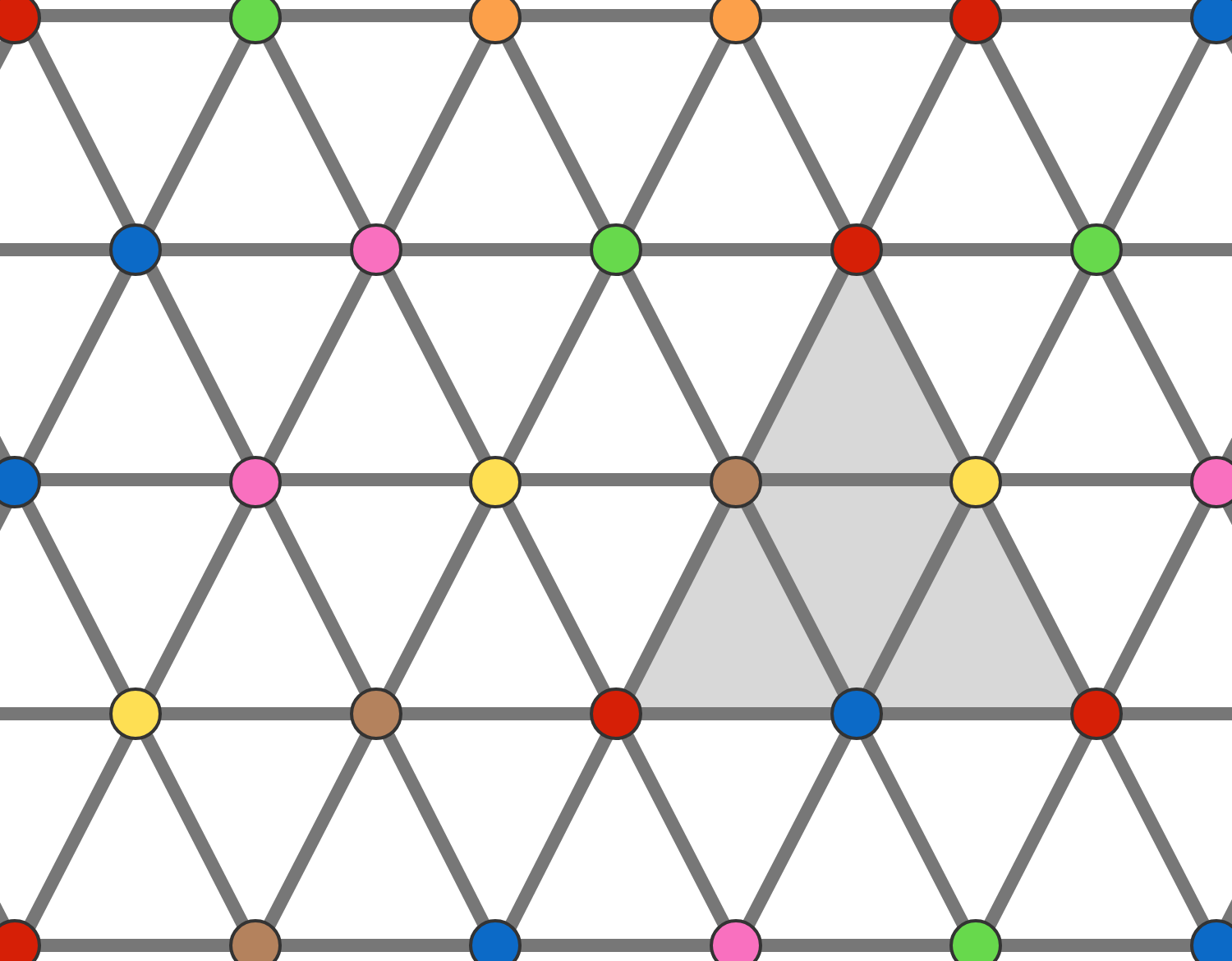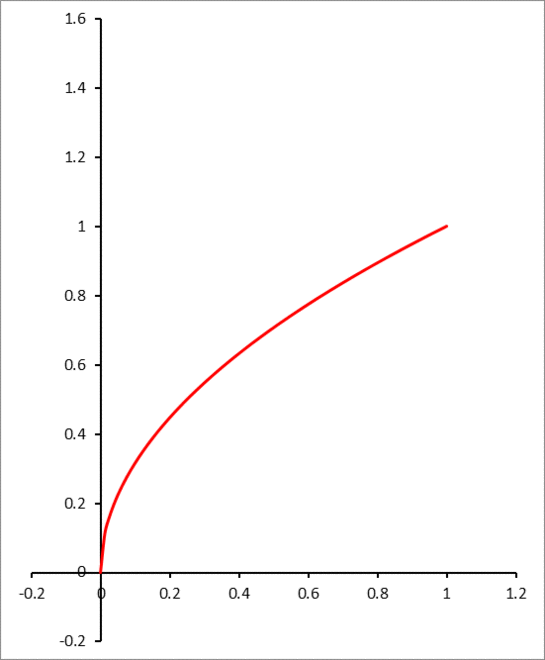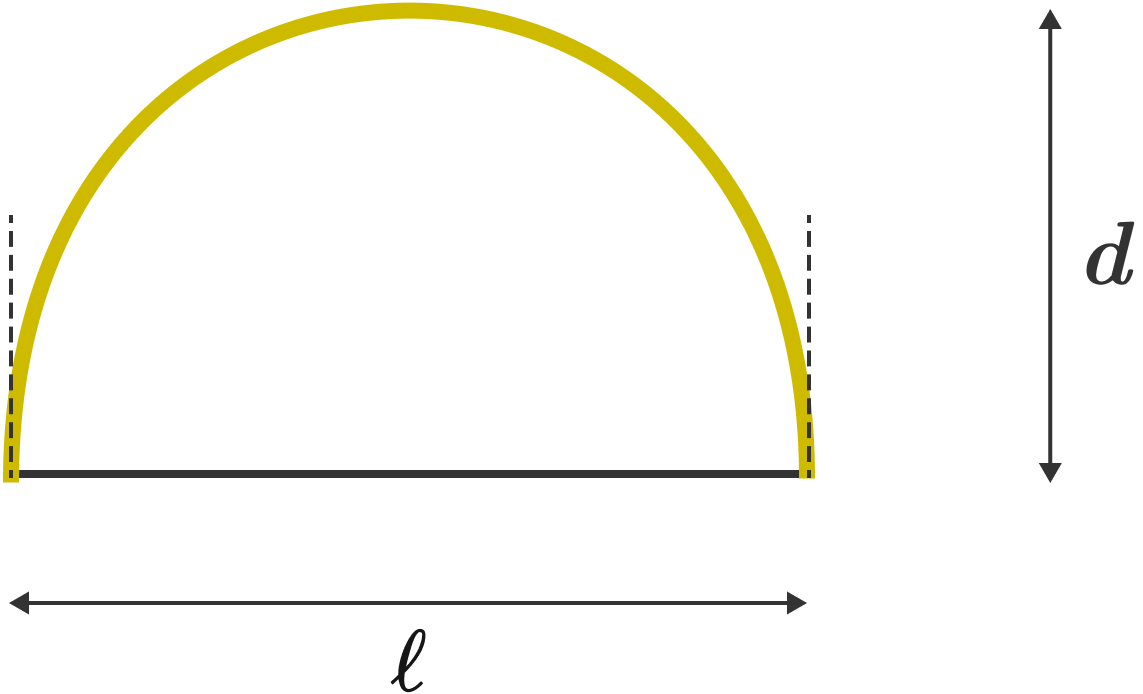# Problems of the Week

Contribute a problemThree triangles of equal perimeters are put together to form a bigger triangle of side lengths 13, 14, and 15.

What is the ratio of the areas of the three triangles?

You have an infinite lattice of equilateral triangles and you would like to fill each node with one of seven colors.

Is there any way to do this so that no equilateral triangle of any size formed by the lattice lines has its three vertices sharing the same color?

For example, the coloring shown would be illegal since the three vertices of the grey triangle share the same color, red.Inspiration

What is the smallest positive integer $N$ such that $\displaystyle \sum_{k=1}^{N}k^{2018}$ is divisible by 2018?

Enter 0 if you come to the conclusion that no such $N$ exists.

The graph of $f(x) = \sqrt{x}$ over the interval $[0, 1]$ is rolled on the $y$-axis.

What are the destination coordinates $(x_0, y_0)$ of the lower tip, which was originally at $(0, 0)?$

Submit $\big\lfloor 1000(x_0+y_0) \big\rfloor$ as your answer.We tighten the black string of the bow until the bow's two ends are parallel, as indicated by the two dotted lines.

The distance between the string and the middle point of the bow is $d,$ and the distance between the two ends is $\ell.$

What is the ratio $\frac{d}{\ell}?$

Assume that the bow is made of a material of uniform circular cross-section of radius $r$ with $r\ll \ell.$ Before tightening the string, the bow was straight.

×